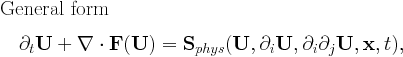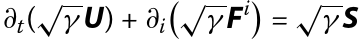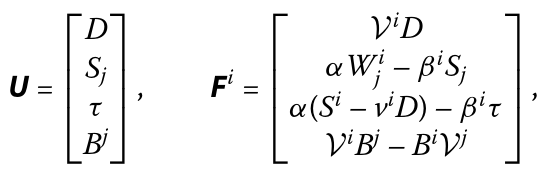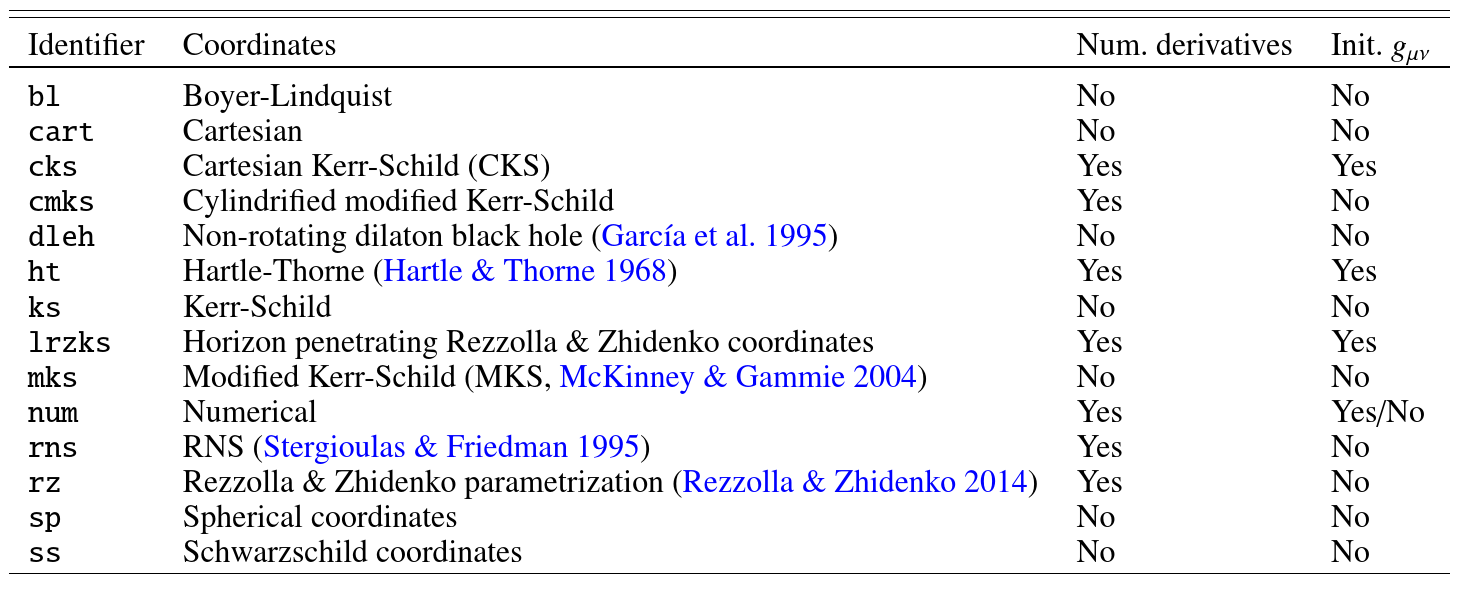# PHYSICS MODULES

This document describes the equations implemented in the src/EQUATION/amrvacphys.t and src/EQUATION/amrvacpar.t files, and gives tips on how a new module can be created. Information about USER defined source terms are in amrvacusr. In principle, the code handles anything of generic formThe code is configured to use the specified equation with the setting

`\$BHAC_DIR/setup.pl -p=EQUATION`
where EQUATION is one of the implemented physics modules (rmhd,...), see below.

## Equation: GRMHD

`\$BHAC_DIR/setup.pl -p=rmhd`
Sets up the following system of equationswith the vector of conserved variables and the sourcesThe parameters for this physics module are `eqpar(gamma_), eqpar(m_), eqpar(a_)` which signify the adiabatic index, the mass-scale (defaulted to 1) and the spin-parameter of the metric (defaulted to 0) respectively.

A detailed discussion of the terms in the conservation law and implementation details can be found in Porth et al. (2017).

The metric can be selected via

`\$BHAC_DIR/setup.pl -coord=METRIC`
and we currently support the following spacetimes (where Identifier=METRIC in the line above)Some of the coordinates allow extra parameters besides mass and spin. These are set via the `coordpar(...)` arrays. We refer to the respective implementations found in `src/geometry/mod_coord_METRIC.t` for details.

Creating a new spacetime is very easy. In principle all BHAC needs are implementations of the subroutines `get_alpha, get_beta, get_g_component` in a corresponding module `src/geometry/mod_coord_METRIC.t`. For convenience, several coordinate transformations should however also be implemented in the module. See e.g. the minimal example of spherical coordinates in `src/geometry/mod_coord_sp.t` for details.

## Creating a New EQUATION Module

A new physics module should be created when the equations to be solved differ significantly from the equations already implemented. If the difference can be described by source terms (e.g. heat conduction, viscosity, radiative cooling, external gravitational field etc.), the specialsource subroutine of the AMRVACUSR module should be used instead of writing a new AMRVACPHYS module.

A new AMRVACPHYS module may be created gradually. The first step is to create a new folder src/NEWEQ with the files amrvacphys.t and amrvacpar.t. You best copy and edit an existing AMRVACPHYS module similar to the new one. The idea is that some functionality (like any solver which requires more info than a maximal physical propagation speed, i.e. anything beyond TVDLF) should only be added at a later stage. In principle, the needed subroutines are at the minimum

```subroutine conserve          (define the primitive to conservative transformation)
subroutine primitive         (define the conservative to primitive transformation)
subroutine getv              (define the advection speed, to be seen in combination with getflux)
subroutine getcmax           (return the (minimal and) maximal physical propagation speed)
subroutine getflux           (define the physical fluxes for all variables)
subroutine addgeometry       (in case other than cartesian cases are needed: geometrical source additions)
```

If your equations contain more restrictions on the time step than the CFL condition, the getdt subroutine could be implemented. For geometric sources, the subroutine addgeometry should provide the geometrical source terms, while other source terms may be given in addsource.

At this point you can use the TVDLF and HLL schemes.

If you think that your new AMRVACPHYS module is of general interest, please send it to the developers, so we may actually make it part of the future release.